# Categories

1

A multiplication is given below where each letter stands for a single number and no two numbers are represented by the same letter.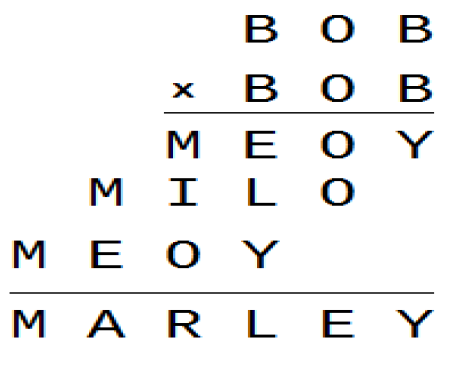What is the value of 2B + M?

#### Explanation:View 10 More CommentsRamanjaneyulu s

Thank youSudhakar

Thans For Giving SolutionPavan Jaiswal

This Solution is very simple thanks for giving video solution.its very eay for understood.Ramanjaneyulu s

Thank youSudhakar

Thans For Giving SolutionPavan Jaiswal

This Solution is very simple thanks for giving video solution.its very eay for understood.

2

A multiplication is given below where each letter stands for a single number and no two numbers are represented by the same letter.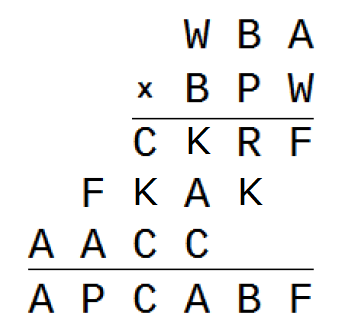What is the value of  P + A + C + K?View 10 More CommentsRamanjaneyulu s

Thank youSudhakar

Thans For Giving SolutionPavan Jaiswal

This Solution is very simple thanks for giving video solution.its very eay for understood.Ramanjaneyulu s

Thank youSudhakar

Thans For Giving SolutionPavan Jaiswal

This Solution is very simple thanks for giving video solution.its very eay for understood.

3

A multiplication is given below where each letter stands for a single number and no two numbers are represented by the same letter.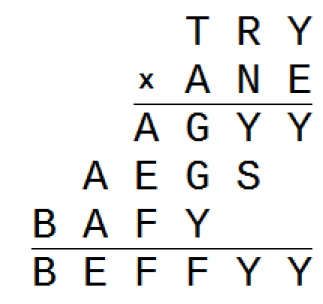Which of the following set forms right angle triangle?View 10 More CommentsRamanjaneyulu s

Thank youSudhakar

Thans For Giving SolutionPavan Jaiswal

This Solution is very simple thanks for giving video solution.its very eay for understood.Ramanjaneyulu s

Thank youSudhakar

Thans For Giving SolutionPavan Jaiswal

This Solution is very simple thanks for giving video solution.its very eay for understood.

4

A multiplication is given below where each letter stands for a single number and no two numbers are represented by the same letter.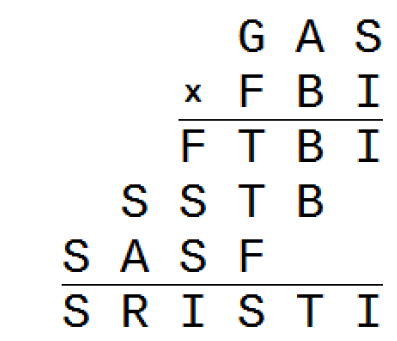Find the value of S + R + I + S + T + I?View 10 More CommentsRamanjaneyulu s

Thank youSudhakar

Thans For Giving SolutionPavan Jaiswal

This Solution is very simple thanks for giving video solution.its very eay for understood.Ramanjaneyulu s

Thank youSudhakar

Thans For Giving SolutionPavan Jaiswal

This Solution is very simple thanks for giving video solution.its very eay for understood.

5

A multiplication is given below where each letter stands for a single number and no two numbers are represented by the same letter.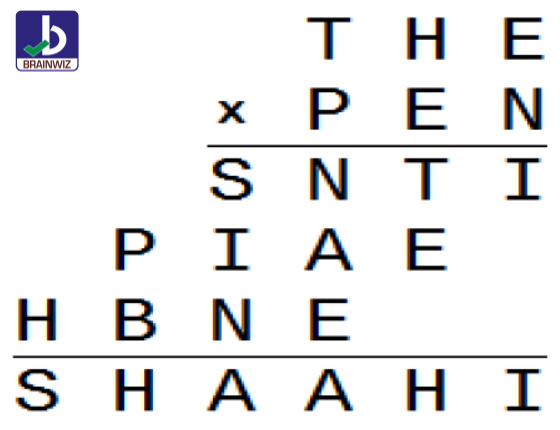What is the value of S + H + A + A + H + I?View 10 More CommentsRamanjaneyulu s

Thank youSudhakar

Thans For Giving SolutionPavan Jaiswal

This Solution is very simple thanks for giving video solution.its very eay for understood.Ramanjaneyulu s

Thank youSudhakar

Thans For Giving SolutionPavan Jaiswal

This Solution is very simple thanks for giving video solution.its very eay for understood.